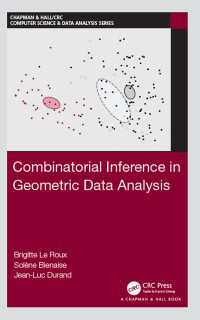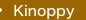•### 幾何学的データ解析における組合せ論的推論Combinatorial Inference in Geometric Data Analysis

• 言語：ENG
• ISBN：9781498781619
• eISBN：9781351651332

ファイル： /

### Description

Geometric Data Analysis designates the approach of Multivariate Statistics that conceptualizes the set of observations as a Euclidean cloud of points. Combinatorial Inference in Geometric Data Analysis gives an overview of multidimensional statistical inference methods applicable to clouds of points that make no assumption on the process of generating data or distributions, and that are not based on random modelling but on permutation procedures recasting in a combinatorial framework.

It focuses particularly on the comparison of a group of observations to a reference population (combinatorial test) or to a reference value of a location parameter (geometric test), and on problems of homogeneity, that is the comparison of several groups for two basic designs. These methods involve the use of combinatorial procedures to build a reference set in which we place the data. The chosen test statistics lead to original extensions, such as the geometric interpretation of the observed level, and the construction of a compatibility region.

Features:

• Defines precisely the object under study in the context of multidimensional procedures, that is clouds of points
• Presents combinatorial tests and related computations with R and Coheris SPAD software
• Includes four original case studies to illustrate application of the tests
• Includes necessary mathematical background to ensure it is self–contained

This book is suitable for researchers and students of multivariate statistics, as well as applied researchers of various scientific disciplines. It could be used for a specialized course taught at either master or PhD level.

### Table of Contents

Introduction

Cloud of Points in a Geometric Space

Combinatorial Typicality Test

Geometric Typicality Test

Homogeneity Permutation Tests

Research Case Studies

Mathematical Bases